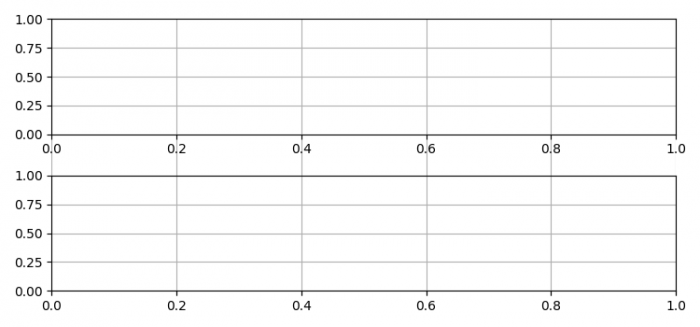# Plotting grids across the subplots in Python Matplotlib

To plot grids across the subplots in Python Matplotlib, we can create multiple subplots and set the spine visibility false out of multiple axes.

## Steps

• Set the figure size and adjust the padding between and around the subplots.
• Create a figure and a set of subplots using subplots() method.
• Add a subplot to the current figure and set its spine visibility as false.
• Turn off the a☓3 labels.
• Share the X-axis accordingly.
• Configure the grid lines for a☓1, a☓2 and a☓3.
• To display the figure, use show() method.

## Example

import matplotlib.pyplot as plt
plt.rcParams["figure.figsize"] = [7.50, 3.50]
plt.rcParams["figure.autolayout"] = True

fig, (ax1, ax2) = plt.subplots(nrows=2)

for _, spine in ax3.spines.items():
spine.set_visible(False)

ax3.tick_params(labelleft=False, labelbottom=False, left=False, right=False)
ax3.get_shared_x_axes().join(ax3, ax1)
ax3.grid(axis="x")

ax1.grid()
ax2.grid()

plt.show()

## OutputUpdated on: 09-Jun-2021

5K+ Views